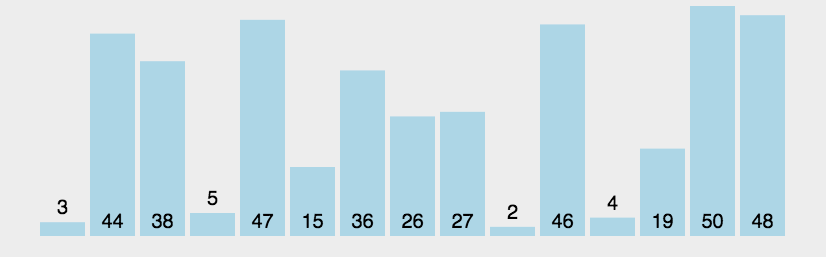# 冒泡排序 ​

## 算法步骤 ​

1. 冒泡排序会比较所有相邻的元素
2. 从第一个数开始，每两个相邻的数进行比较
3. 这两个相邻的数比较完后，按照小的放在左边，大的放在右边的想法，交换它们的位置
4. 执行完一轮后，大的数就会被排到最右边
5. 反反复复重复 n - 1 遍后，整个排序就完成了

## 动画演示链接 ​

https://visualgo.net/zh/sorting## 基础案例 ​

• 时间复杂度：O (n ^ 2)
• 空间复杂度：O (1)
javascript
``````Array.prototype.bubbleSort = function () {
for (let i = 0; i < this.length - 1; i++) {
for (let j = 0; j < this.length - 1 - i; j++) {
if (this[j] > this[j + 1]) {
const temp = this[j]
this[j] = this[j + 1]
this[j + 1] = temp
}
}
}
}

const arr = [5, 4, 3, 2, 1]

arr.bubbleSort() // [1, 2, 3, 4, 5]``````
``````Array.prototype.bubbleSort = function () {
for (let i = 0; i < this.length - 1; i++) {
for (let j = 0; j < this.length - 1 - i; j++) {
if (this[j] > this[j + 1]) {
const temp = this[j]
this[j] = this[j + 1]
this[j + 1] = temp
}
}
}
}

const arr = [5, 4, 3, 2, 1]

arr.bubbleSort() // [1, 2, 3, 4, 5]``````